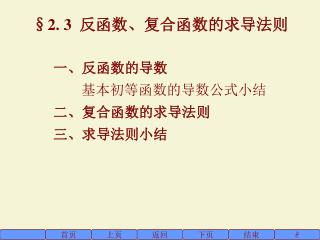Download Presentation§2. 3 反函数、复合函数的求导法则§2. 3 反函数、复合函数的求导法则 - PowerPoint PPT Presentation

Download Presentation§2. 3 反函数、复合函数的求导法则
An Image/Link below is provided (as is) to download presentation

Download Policy: Content on the Website is provided to you AS IS for your information and personal use and may not be sold / licensed / shared on other websites without getting consent from its author. While downloading, if for some reason you are not able to download a presentation, the publisher may have deleted the file from their server.

- - - - - - - - - - - - - - - - - - - - - - - - - - - E N D - - - - - - - - - - - - - - - - - - - - - - - - - - -
Presentation Transcript

1. §2. 3 反函数、复合函数的求导法则 一、反函数的导数 基本初等函数的导数公式小结 二、复合函数的求导法则 三、求导法则小结 首页 上页 返回 下页 结束 

2. 一、反函数的导数 如果函数x=j(y)在某区间Iy内单调、可导且j(y)0，那么它的反函数y=f(x)在对应区间Ix内也可导，并且 简要证明： 因为y=f(x)连续，所发当Dx0时，Dy0。 下页

3. 一、反函数的导数 如果函数x=j(y)在某区间Iy内单调、可导且j(y)0，那么它的反函数y=f(x)在对应区间Ix内也可导，并且 例1．求(arcsin x)及(arccos x)。 解： 因为y=arcsin x是x=sin y的反函数，所以 下页

4. 一、反函数的导数 如果函数x=j(y)在某区间Iy内单调、可导且j(y)0，那么它的反函数y=f(x)在对应区间Ix内也可导，并且 例2．求(arctan x)及(arccot x)。 解： 因为y=arctan x是x=tan y的反函数，所以 下页

5. 基本初等函数的导数公式小结： (1) (C)=0， (2) (xm)=mxm-1， (3) (sin x)=cos x， (4) (cos x)=-sin x， (5) (tan x)=sec2x， (6) (cot x)=-csc2x， (7) (sec x)=sec x tan x， (8) (csc x)=-csc x cot x， (9) (ax)=axln a ， (10) (ex)=ex， 上页

6. 二、复合函数的求导法则 如果u=j(x)在点x0可导，函数y=f(u)在点u0=j(x0)可导，则复合函数y=f[j(x)]在点x 0可导，且其导数为 简要证明： 假定u=j(x)在x0的某邻域内不等于常数，则Du0，此时有 =f(u 0)j(x 0)。 下页

7. 二、复合函数的求导法则 如果u=j(x)在点x0可导，函数y=f(u)在点u0=j(x0)可导，则复合函数y=f[j(x)]在点x 0可导，且其导数为 如果 u=j(x)在开区间 Ix内可导，y=f(u)在开区间 Iu内可导，且当xIx时，对应的uIu，那么复合函数y=f[j(x)]在区间Ix内可导，且下式成立： 下页

8. 复合函数的求导法则： 解：函数y=lntan x是由y=ln u，u=tan x复合而成， 下页

9. 复合函数的求导法则： 对复合函数求导法则比较熟练以后，就不必再写出中间变量。 下页

10. 复合函数的求导法则： 复合函数求导法则可以推广到多个函数的复合。 下页

11. 复合函数的求导法则： 解：y=(sin nx) sinnx + sin nx (sinnx) =ncos nxsinnx+sin nxn sinn-1x(sin x ) =ncos nxsinnx+n sinn-1x cos x =n sinn-1x sin(n+1)x。 上页

12. 三、求导法则小结 函数的和、差、积、商的求导法则： (1) (uv)=uv， (2) (Cu)=Cu (C是常数)， (3) (uv)=uv+uv， 反函数求导法： 复合函数的求导法则： 结束